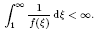Home

Blow-up of solutions of a quasilinear parabolic equation

Abstract

We consider non-negative solutions of the Cauchy problem for quasilinear parabolic equations ut = Δum + f(u), where m > 1 and f(ξ) is a positive function in ξ > 0 satisfying f(0) = 0 and a blow-up conditionWe show that if ξm+2/N /(−log ξ)β = O(f(ξ)) as ξ ↓ 0 for some 0 < β < 2/(mN + 2), one of the following holds: (i) all non-trivial solutions blow up in finite time; (ii) every non-trivial solution with an initial datum u0 having compact support exists globally in time and grows up to ∞ as t → ∞: limtt→∞ inf|x|<Ru(x, t) = ∞ for any R > 0. Moreover, we give a condition on f such that (i) holds, and show the existence of f such that (ii) holds.

Metrics

Full text viewsFull text views reflects the number of PDF downloads, PDFs sent to Google Drive, Dropbox and Kindle and HTML full text views.

Total number of HTML views: 0
Total number of PDF views: 0 *Loading metrics...

Abstract viewsAbstract views reflect the number of visits to the article landing page.

Total abstract views: 0 *Loading metrics...

* Views captured on Cambridge Core between <date>. This data will be updated every 24 hours.

Usage data cannot currently be displayed